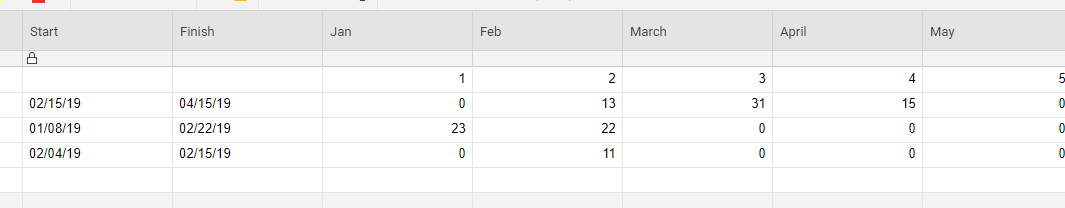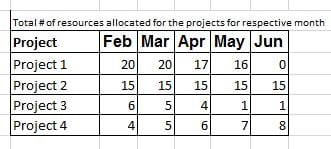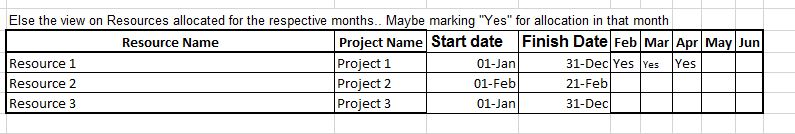# Sumifs with Date Range

Hi there!

I'm using Smartsheet to keep track of timelines and allocated ressources called FTEs across multiple projects. I'm trying to figure otut a way using the SUMIFS formula to sum up the usage of FTEs across all projects per month.

Currently, I'm using

=SUMIFS(FTE:FTE; Projekt:Projekt; "Project A"; Start:Start; <=DATE(2019; 01; 31); End:End; >=DATE(2019; 01; 31))

This will sum up all the FTEs in column FTE that correspond to Project A in January just fine. The problem is with months that are not fully covered, say a preoject only lasts until 15/02/2019. This will count the full february and thus suggest higher FTE usage than really required. Any way around this?

Best wishes, Alex

«1

• Have you tried factoring in the DAY function to try to pull a percentage of the month (almost like prorating which can also be done in SS)?

=DAY([End Date Column]@row) / DAY(DATE(YEAR([End Date Column]@row), MONTH([End Date Column]@row) + 1, 1) - 1)

This will give you a decimal (one day is approximately .035) based off of how much of the month was actually worked. You may be able to factor this in somehow to get more accurate counts.

Actually I have tried the formula, but I only get numbers out that I do not understand, e.g. one month that ends on the 25th provides the number 0,80645, devided by 0,035 =23 not 25..

What I would need is some formula to disect a duration from 15/01 until 15/03 into

January: 17 days

February: 28 days

March: 15 days

Then I can easily summ um the days per project per month and multiply with my ressouring...

Is that possible?

Thanks a lot, Alex

• It is possible. I found the easiest way was to build a table as in the screenshot below. In the Jan2 cell, enter the following formula:

.

=IF(AND(Jan\$1 = MONTH(\$Start@row), Jan\$1 = MONTH(\$Finish@row)), DAY(\$Finish@row) - DAY(\$Start@row), IF(Jan\$1 < MONTH(\$Start@row), 0, IF(MONTH(\$Start@row) = Jan\$1, DAY(IFERROR(DATE(YEAR(\$Start@row), Jan\$1 + 1, 1), DATE(YEAR(\$Start@row) + 1, 1, 1)) - 1) - DAY(\$Start@row), IF(AND(Jan\$1 > MONTH(\$Start@row), Jan\$1 < MONTH(\$Finish@row)), DAY(IFERROR(DATE(YEAR(\$Start@row), Jan\$1 + 1, 1), DATE(YEAR(\$Start@row) + 1, 1, 1)) - 1), IF(Jan\$1 = MONTH(\$Finish@row), DAY(\$Finish@row), IF(Jan\$1 > MONTH(\$Finish@row), 0))))))

.

You can then dragfill across and down as needed. Here's a basic breakdown of what happens...

1. If the Start and Finish Months both equal the corresponding month column, it will just subtract the days and give you the difference. (Feb4)

2. If the Start Month is greater than the corresponding month column, it will display 0 to show that no days were worked in that month. (Jan2)

3. If the Start Month is the same as the corresponding month column, it will...

3.a. Go to the First of the next month and then subtract a day. This ensures you will always be calculating from the proper number of days regardless of month. In the case of December (the IFERROR section), it will go to the first day of the first month of the next year and then subtract a day.

3.b. It will then subtract the starting day from the last day of the month to display how many days were worked in that first month. (Feb2)

4. If the corresponding month column is greater than the Start Month but less than the Finish Month, it will use a similar calculation as in step 2 to pull the last day of the month (meaning the entire month was worked). (March2)

5. If the Finish Month is the same as the corresponding month column, it will simply pull the day from the finish date as that will be how many days in that month were worked. (April2)

6. And finally... If the Finish Month is less than the corresponding month column, then there were no days worked in that month, and the formula will give you a 0. (May2)• Hi Paul!

Great, that seems to do the trick!

However, it only works within one year. I have projects that start in october and end in march of the next year. Would it be possible to add the year as well?

Thanks yo so much!

Alex

• Yes. How were you wanting it displayed? Something along the lines of...

.

Oct 18     Nov 18      Dec 18      Jan 19      Feb 19       March 19

.

or would you prefer to only show what was done in 2019 and have the 2018 data elsewhere? Maybe a 2018 table and a 2019 table and then compare the two to show overlap?

• Hi Paul!

Something like

Oct18  Nov18 Dec18 Jan19 Feb19

would be totally awsome! I will need to have 3 years visible at a glance, so I would prefer to have it all in one sheet!

Thank you so much!

• Take a glance at this mess. Here's a published link to the sheet (currently read only).

.

And here's the formula used (with column and row references locked to make dragfill/autofill work easily)

=IF(AND(\$Start@row >= DATE([Month 1]\$2, [Month 1]\$1, 1), \$Finish@row <= IFERROR(DATE([Month 1]\$2, [Month 1]\$1 + 1, 1), DATE([Month 1]\$2 + 1, 1, 1)) - 1), \$Finish@row - \$Start@row, IF(AND(MONTH(\$Start@row) = [Month 1]\$1, YEAR(\$Start@row) = [Month 1]\$2), (IFERROR(DATE([Month 1]\$2, [Month 1]\$1 + 1, 1), DATE([Month 1]\$2 + 1, 1, 1)) - 1) - \$Start@row, IF(AND(\$Start@row < DATE([Month 1]\$2, [Month 1]\$1, 1), \$Finish@row > IFERROR(DATE([Month 1]\$2, [Month 1]\$1 + 1, 1), DATE([Month 1]\$2 + 1, 1, 1)) - 1), DAY(IFERROR(DATE([Month 1]\$2, [Month 1]\$1 + 1, 1), DATE([Month 1]\$2 + 1, 1, 1)) - 1), IF(AND(MONTH(\$Finish@row) = [Month 1]\$1, YEAR(\$Finish@row) = [Month 1]\$2), DAY(\$Finish@row)))))

.

To get the Total Days Worked, I used a basic

=SUMIFS([Month 1]@row:[Month 36]@row, [Month 1]@row:[Month 36]@row, ISNUMBER(@cell))

• WOW!

Super! Works like a charm!

Thank yo so much for your help!

Best regards, Alex

• Thanks.. this is wonderful formula which helped me aswell. Though just wanted to check if we can get just an some value which will indicate that the FTE was allocated in that month.

To explain.. Maybe the allocation is between Jan 2019 till Mar 2019... it should just Indicate "Y" for the allocated months - Jan, Feb and Mar. For Apr it can be blank row.

• Could you provide some screenshots of what exactly you are looking to accomplish?

• Thanks for the response Paul

Actually looking for data as per the screenshot 1. this is just count of resources assigned on the project for the respective months.

If the above is not possible then the screenshot 2 - which is indicating the resource allocation for the specific month.• You will want a similar layout to the above solution, but do not need the start and finish dates. You will put the month numbers across row 1 and the year in row one of the resource name column. I will assume it is on the same sheet as the data.

The formula to put in Jan2 would be

=COUNTIFS(\$Resource:\$Resource, \$[Resource Name]@row, \$[Start Date]:\$[Start Date], MONTH(@cell) >= Jan\$1, \$[Finish Date]:\$[Finish Date], MONTH(@cell) <= Jan\$1)

You would then drag-fill down and over, and you should be set.

• Thanks, though how can i count for the specific projects as in the screenshot 1.

I just want the total monthly resources count on the specific project.. month on month

• My apologies. I was multitasking (but obviously not very well...

Project Table    Jan     Feb     Mar     Apr     May     June

2019           1        2         3         4         5           6

A              F

B

C

.

If your table is set up above, you would use a formula similar to below (just change column names as needed) and put it where you see the "F".

=COUNTIFS(\$[Project Column]:\$[Project Column], \$[Project Table]@row, \$[Start Date]:\$[Start Date], MONTH(@cell) >= Jan\$1, \$[Finish Date]:\$[Finish Date], MONTH(@cell) <= Jan\$1)

This will look down the Project column in your master list (assuming every row is a separate resource with a project assigned) and count how many times it finds the project name that is in the Project Table column for whatever row the formula is on and within the specified month for whatever column it is in.

• Thank you for the response. I will try and get back to you..

## Help Article Resources

Want to practice working with formulas directly in Smartsheet?

Check out the Formula Handbook template!